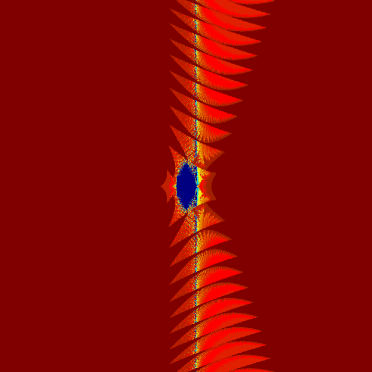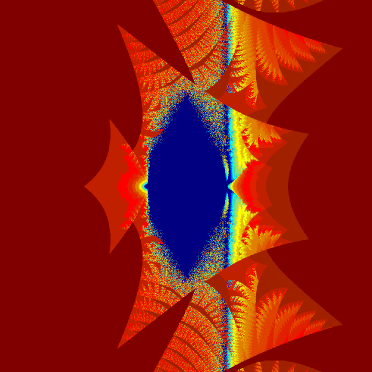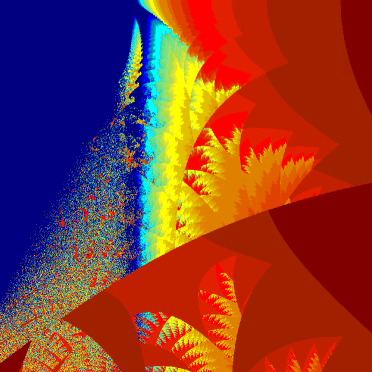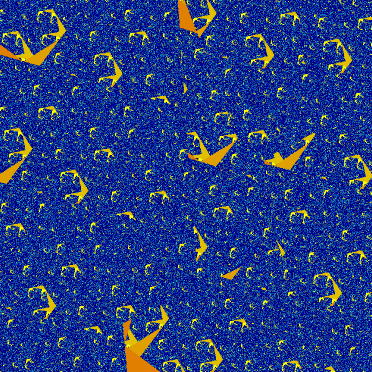# Fractal Fun

A couple of years ago I wrote a post where I talked about taking the imaginary number i to it’s own power an infinite amount of times, essentially

$$\LARGE{i^{i^{i^{i^{.^{.^.}}}}}}$$.

I showed numerically at least that it converges upon a single value in the complex plane, but then I speculated about what would happen if I did the same for other numbers? I thought it would most likely be a fractal like the Mandelbrot set or the Julia set, but I never got around to actually solving it.

Well, for the class that I’m TA’ing this semester (intro to computing for Chemical Engineers), I got hold of some simple code for making the Mandelbrot set in MATLAB to show the students a fun and interesting example of using for loops. After I did that though, I figured that it would be fairly simple to modify the code to see if taking a complex number to it’s own power created a fractal or not. So my algorithm was the following:

For any point c in the complex plane, let
$$\displaystyle{z_0=c}$$
$$\displaystyle{z_{n+1}={z_n}^c}$$.
In the limit as n goes to infinity, if z remains finite, then c is within the set. Otherwise it is not in the set.

Since you can’t really evaluate it to infinity, what you do instead is implement an algorithm where you perform the iteration a large but finite number if times, and see if the iteration stays inside a set radius. So here is my fractal. The first plot shows the the complex plane from -20 to +20 on both the real and imaginary axis:Here the blue football-shaped region in the center is centered at the origin. These points are considered to be ‘within’ the set, since a large number of iterations did not give numbers that were outside of the convergence radius (I set the number of iterations to 50, and the convergence radius to 1000). Any points that are not that deep blue are considered to be ‘outside’ the set, with the color being an indication of how many iterations it took for that point to leave the convergence radius. The dark red points took the least iterations, with the orange, yellow, green, and light blue areas taking progressively more iterations.

I think the feathery wing structures going in the positive and negative imaginary directions are really interesting. They continue up and down seemingly without end, or at least I didn’t see any end for even large plots that I made. This is in sharp contrast to the Mandelbrot and Julia sets which are confined to a finite area.

But the center looks the most interesting, so let’s get a closeup of that.There’s a lot of interesting stuff going on in this area, with some really beautiful feathery wings, and a jumbled area that just looks like ‘static’. Here’s a zoom-in that let’s us see a little bit of both better.Wow. Those feathers really are quite beautiful if I do say so myself. That random static-looking area bugs me though, since random noise really isn’t a feature of fractals. Instead they exhibit more of an ‘ordered chaos’ showing infinite complexity. So for my last image here is a much closer zoom on the static-looking area.This last image has a width and height of just 0.005. Here we can see that it really is infinitely complex and not just random noise. I did another with a width and height of 0.0005, and it looks very similar, so there is some degree of self-similarity, much like we see in other fractals.

So the only thing this set is missing is a name. In reality probably someone has calculated this set and given it a name, but on the slight chance that no one has, I hereby name it the Bassett set. (How’s that for hubris? I bet Benoit Mandelbrot didn’t name the set after himself, but that someone else named it after him in honor of his discovery)

This entry was posted in Science. Bookmark the permalink.

This site uses Akismet to reduce spam. Learn how your comment data is processed.